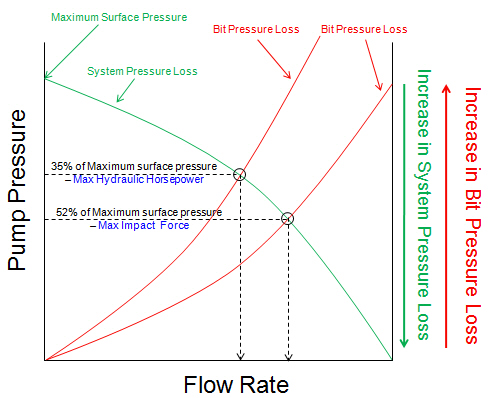## Pressure Losses Through Annulus

Pressure drop in the annulus is another important part of drilling hydraulic calculation and today we would like to demonstrate the equations specifically used to determine pressure loss in the annulus.The following equations are used for calculating pressure loss in the annulus.## Coefficient For ID of Pipe and Tool Joints

You’ve learned about pressure loss in the drill string from the previous topic (Pressure Losses through Drill String Calculation). The general coefficient for internal diameter of pipe does not account the inside diameter of tool joint.

The general coefficient equation is shown below:Coefficient of The Bore of Drill Pipe and Tool Joint

To get the accurate pressure loss calculation, the coefficient of a section of pipe must be accounted for both the pipe ID and the tool joint ID.In general, each 100 ft of pipe, there is appoximately 6.7 ft of tool joint length (6.7% of total length) and 93.3 ft of drill pipe (93.3% of total length). Therefore, the general coefficient for internal diameter of pipe can be derived in to this following equation: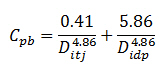## Pressure Losses Through Drill String Calculation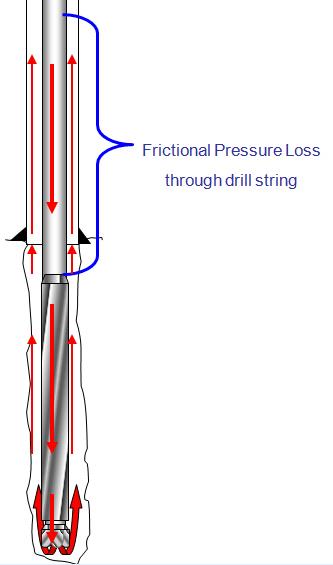To determine pressure drop while the drilling mud is passing through a circular pipe (drill pipe and drill collar), you can calculate pressure loss with the following equation: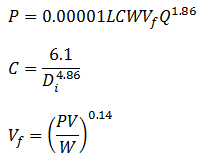Assumptions:

Turbulent viscosity = 3 cp

Friction factor = 0.378(Q/D)-0.14

The first equation has a coefficient (C) to take into account the friction and the geometry of the bore ID.

Where:

P = pressure loss, psi

L = length of pipe, ft

W = mud density, ppg

Vf = viscosity correction factor

PV = plastic viscosity, centipoises

Q = flow rate, gpm

Di = inside diameter of pipe, inch

C = general coefficient for internal diameter of pipe

## Drilling Hydraulics Optimization for the System with Downhole Tools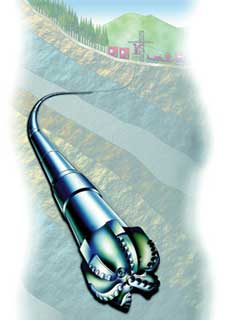BHA - Ref. Spe.org

The system with downhole tools means the drill string consisting of drill pipe, drill collar, down hole equipment (MWD, PWD, LWD) and a bit. The process of optimization this system is more complicated than the simple system. Due to the fact that the down hole equipment responses differently when changing in flow rate, even the similar tool, the pressure responses cannot be modeled with simple equation.

There are two methods used to optimize drilling hydraulics with downhole tools.

1. The first method is to subtract the expected pressure loss generated by downhole tools from the surface pressure. After you get the reduced surface pressure, you optimize the system with lower pump pressure.

2. The second method is to optimize whole system as if there is no downhole equipment and then you subtract the expected pressure loss by downhole tools from available bit pressure.

What are the differences between both methods of optimization with downhole tools?

## Optimize Drilling Hydraulics for the Basic System

The basic system means the drill string consisting of drill pipe, drill collar and a bit (no downhole tools). The process for drilling hydraulic optimization is simple. The following steps demonstrate how to optimize drilling hydraulics for the basic system.

• Select drill pipe and drill collar which provide pressure loss at a minimum for the range of depth and flow rate.

• Decide the methods to optimize drilling hydraulics. If you drill a shallow hole section, the maximum impact force is recommended. However, if you drill a deeper section, the maximum hydraulic horsepower is better than the maximum impact force.

• Determine flow rate to meet your optimization requirement at the end of the bit run. The curve below gives you a idea how you can get the flow rate from the char at each particular optimization method.